## Welcome to the World of Modelling and Simulation

### What is Modelling?

This blog is all about system dynamics modelling and simulation applied in the engineering field, especially mechanical, electrical, and ...### Finite Element Analysis with Abaqus: Part 3 - Reinforced Concrete Frame Stress Analysis

This is a continuation of the original topic "Finite Element Analysis with Abaqus". This tutorial demonstrates how to solve the stress distribution of a reinforced concrete frame. The following figure depicts a reinforced frame, which has two three dimensional beams and two three dimensional columns.

The finite element model development and solution are divided into the following steps:

1. 3D model development
1.1 Creating the geometry
1.2 Selecting the material

2. Creating the mesh

3. Setting up the initial and boundary conditions

4. Frame stress/strain analyses
4.1 Results and Discussions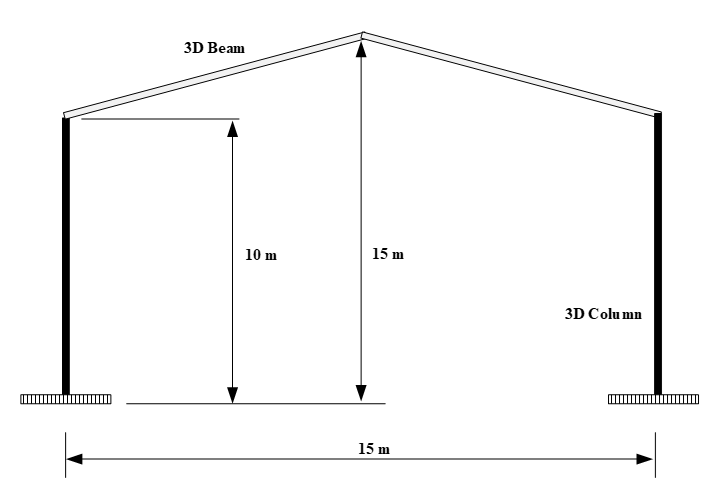Fig. 1: Showing a 3D reinforced concrete frame.

1. 3D model development

In Abaqus CAE, the geometry is modelled into four parts: two individual 3D beams and two individual 3D columns. The column dimension is 36 cm by 51 cm.

The cross section of the beams is 25 cm x 25 cm. The uniformly distributed load acting on the frame top is 64 KN/m.

1.1 Creating the geometry

We, first, create the 3D geometry for each beam and each column in the CAD environment of Abaqus, which is depicted below.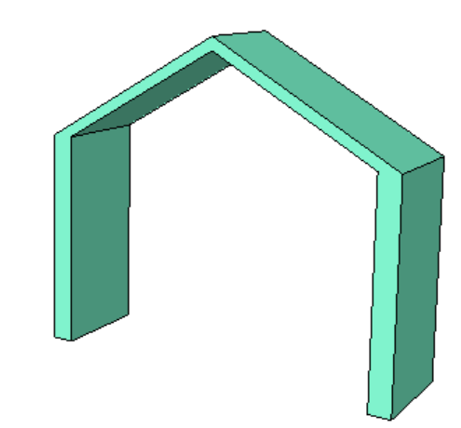Fig. 2: Showing the 3D CAD geometry of the reinforced concrete frame.

1.2 Selecting the material

The mechanical material properties for the 3D reinforced beam panel are chosen as the Young’s modulus and Poisson’s ratio, which are 2000000000 Pa and 0.30, respectively. The material is homogeneous, and the stress-strain ratio is set to 1. In order to assign these properties, sections are created in Abaqus.

2. Creating the mesh

In the second stage of the FE model development, we create the mesh for the geometry that is developed in the earlier stage. We have chosen 3D linear element for the meshing. An 8 node brick element is selected where total 19825 elements are generated. Table 1 documents the mesh information – such as, the convergence and verification of the meshing, number of elements, element types etc.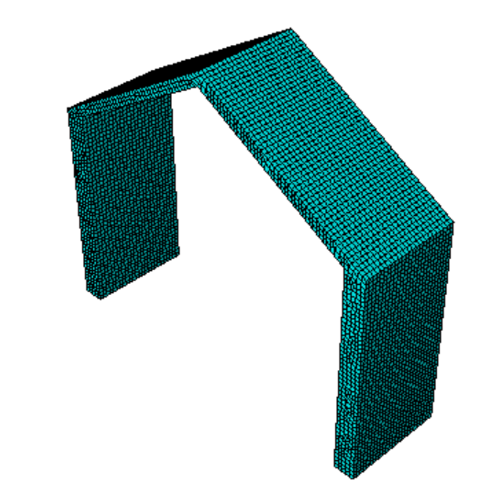Fig. 3: Showing a 3D reinforced concrete frame after meshing is done.

Number of elements :  19825,
Analysis errors:  0 (0%),
Analysis warnings:  0 (0%)

3. Setting up the initial and boundary conditions

For this simulation type, we do not require any initial condition. However, there are some boundary conditions.  We fix the end of the 3D columns. We apply the concentrated loads at each joints of the structure. We also apply the pressure applied to inclined 3D beam.Fig. 4: Showing the loads and boundary conditions acting on the 3D structure.

4. Stress/strain analyses

In this part, we simulate the loads/pressures on the 3D frame and how the stress is distributed to the structure.

4.1 Results and Discussions

The 3D reinforced concrete beam is simulated here. Fig. 4 depicts the loads or forces that are acting on the frames and the boundary conditions. The magnitude of the uniformly distributed pressure on the beam is 60 KN/m.

The following shows the undeformed and deformed meshed frame.Fig. 5: Showing the undeformed (left) and deformed (right) shape of the geometry.

Following Fig. 6 shows the contour plot on the undeformed 3D frame.Fig. 6: Showing the contour plot on the undeformed shape of the frame.

The following Fig. 7 shows the contour plot on the deformed shape of the structure.Fig. 7: Showing the contour plot on the deformed shape of the frame.

Fig. 8 shows the contour stress plot on the deformed-undeformed shape.Fig. 8: Showing the contour plot on the undeformed and deformed shape of the frame.

Fig. 9 shows nodal spatial distribution on the undeformed shape of the reinforced frame.Fig. 9: Showing the strain distribution on the undeformed shape of the frame.

The following result in Fig. 10 shows the in plane principal stresses on the structure on the undeformed shape.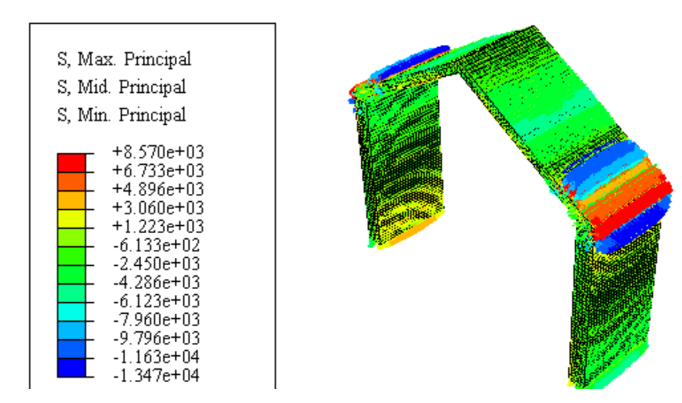Fig. 10: Showing the planar principal stresses on the undeformed shape of the frame.

The following result in Fig. 11 shows the in plane principal stresses on the structure on the deformed shape.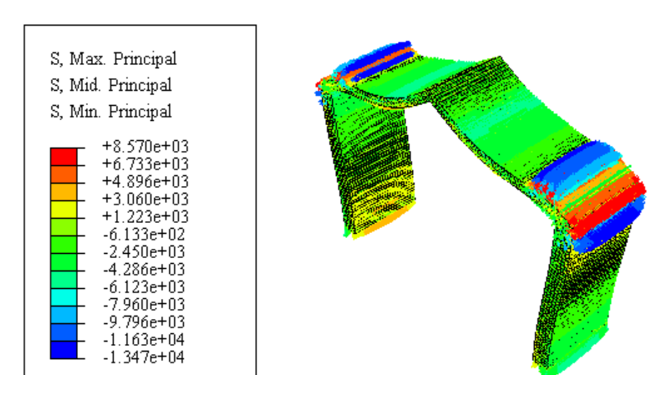Fig. 11: Showing the planar principal stresses on the deformed shape of the frame.

Fig. 12 highlights the in plane principal stresses on the structure on both deformed -undeformed shape.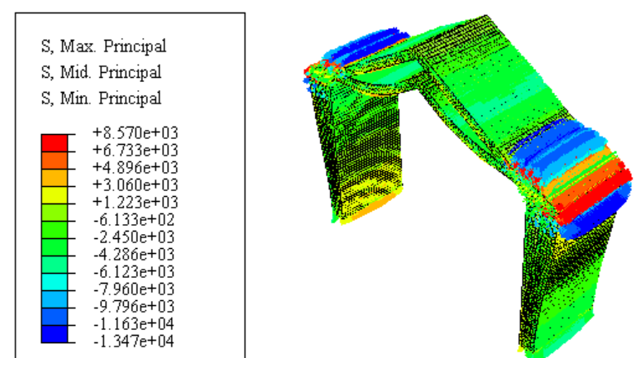Fig. 12: Showing the planar principal stresses on the deformed and undeformed shape of the frame.

Fig.13 shows the strain distribution on the planar stress plot of the geometry.Fig. 13: Showing the spatial nodal distribution on the undeformed shape of the frame.

From the above results, we observe that the stress concentrations are more on the corners or edges of the 3D reinforced structure.

#ConcreteFrameStressAnalysis   #AbaqusCAE  #StressStrainAnalyses   #NodalDistribution   #VonMisesStress